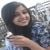# Michael Rosen (mathematician)

Updated on
Covid-19
 Name  Michael RosenAwards  Chauvenet Prize Role  Mathematician Books  A Classical Introduction to Modern Number TheoryPeople also search for  Kenneth Ireland, David Goss, Brian F. Hoffman

Michael Ira Rosen (born 7 March 1938 in Brooklyn) is an American mathematician who works on algebraic number theory, arithmetic theory of function fields, and arithmetic algebraic geometry.

Rosen earned a bachelor's degree from Brandeis University in 1959 and a PhD from Princeton University in 1963 under John Coleman Moore with thesis Representations of twisted group rings. He is a mathematics professor at Brown University.

Rosen is known for his textbooks, especially for the book with co-author Kenneth Ireland on number theory, which was inspired by ideas of Andre Weil; this book A Classical Introduction to Modern Number Theory gives an introduction to zeta functions of algebraic curves, the Weil conjectures, and the arithmetic of elliptic curves.

For his essay Niels Hendrik Abel and equations of the fifth degree Rosen received the Chauvenet Prize.

## Publications

• with Kenneth Ireland: A classical introduction to modern number theory, Springer, Graduate Texts in Mathematics, 1982, 2nd edn. 1992, ISBN 038797329X (Rosen and Ireland earlier published Elements of number theory; including an introduction to equations over finite fields, Bogden and Quigley, 1972)
• Number theory in function fields, Springer, Graduate Texts in Mathematics, 2002, ISBN 0-387-95335-3
• Remarks on the history of Fermat’s last theorem 1844 to 1984, in Cornell, Silverman, Stevens (eds.): Modular forms and Fermat's last theorem, pp. 505–525, Springer, New York 1997.
• Abel’s theorem on the lemniscate, American Mathematical Monthly 88, 1981, pp. 387–395
• Niels Hendrik Abel and equations of the fifth degree, American Mathematical Monthly 1995
• ## References

Similar Topics
David Goss
A Womans Face
Björn Andrae
Topics